# Applied Machine Learning: Module 2 (Supervised Learning, Part I)¶

## Classification and Regression¶

• Both classification and regression take a set of training instances and learn a mapping to a target value
• For classification, the target value is a discrete class value

• Binary: target value 0 (negative class) or 1 (positive class)
• e.g. detecting a fraudulent credit card transaction
• Multi-class: target value is one of a set of discrete values
• e.g. labelling the type of fruit from physical attributes
• Multi-label: there are multiple target values (labels)
• e.g. labelling the topics discussed on a Web page
• For regression, the target value is continuous (floating point / real-valued)

• e.g. predicting the selling price of a house from its attributes
• Looking at the target value's type will guide you on what supervised learning method to use
• Many supervised learning methods have 'flavors' for both classification and regression

## Supervised learning methods: Overview¶

• To start with, we'll look at two simple but powerful prediction algorithms:
• K-nearest neighbors
• Linear model fit using least-squares
• These represent two complementary approaches to supervised learning:
• K-nearest neighbors makes few assumptions about the structure of the data and gives potentially accurate but sometimes unstable predictions (sensitive to small changes in the training data)
• Linear models make strong assumptions about the structure of the data and give stable but potentially inaccurate predictions.

## What is a Model?¶

It's a specific mathematical or computational description that expresses the relationship between a set of input variables and one or more outcome variables that are being studied or predicted. In statistical terms the input variables are called independent variable and outcome variables are termed dependent variables. In machine learning we use the term features to refer to the input, or independent variables, and target value or target label to refer to the output, dependent varbales

## Generalization, Overfitting, and Underfitting¶

• Generalization ability refers to an algorithm's ability to give accurate predictions for new, previously unseen data
• Assumptions:
• Future unseen data (test set) will have the same properties as the current training sets, or more technically, as drawn from the same underlying distribution as the training set.
• Thus, models that are accurate on the training set are expected to be accurate on the test set.
• But that may not happen if the training model is tuned too specifically to the training set.
• Models that are too complex for the amount of training data available are said to overfit and are not likely to generize well to new examples.
• Models that are too simple, that don't even do well on the training data, are said to underfit and also not likely to generlize well

## Preamble and Review¶

In :
%matplotlib notebook
import numpy as np
import pandas as pd
import seaborn as sn
import matplotlib.pyplot as plt

from sklearn.model_selection import train_test_split
from sklearn.neighbors import KNeighborsClassifier

np.set_printoptions(precision=2)

feature_names_fruits = ['height', 'width', 'mass', 'color_score']
X_fruits = fruits[feature_names_fruits]
y_fruits = fruits['fruit_label']
target_names_fruits = ['apple', 'mandarin', 'orange', 'lemon']

X_fruits_2d = fruits[['height', 'width']]
y_fruits_2d = fruits['fruit_label']

X_train, X_test, y_train, y_test = train_test_split(X_fruits, y_fruits, random_state=0)

from sklearn.preprocessing import MinMaxScaler
scaler = MinMaxScaler()
X_train_scaled = scaler.fit_transform(X_train)
# we must apply the scaling to the test set that we computed for the training set
X_test_scaled = scaler.transform(X_test)

knn = KNeighborsClassifier(n_neighbors = 5)
knn.fit(X_train_scaled, y_train)
print('Accuracy of K-NN classifier on training set: {:.2f}'
.format(knn.score(X_train_scaled, y_train)))
print('Accuracy of K-NN classifier on test set: {:.2f}'
.format(knn.score(X_test_scaled, y_test)))

example_fruit = [[5.5, 2.2, 10, 0.70]]
example_fruit_scaled = scaler.transform(example_fruit)
print('Predicted fruit type for ', example_fruit, ' is ',
target_names_fruits[knn.predict(example_fruit_scaled)-1])

Accuracy of K-NN classifier on training set: 0.95
Accuracy of K-NN classifier on test set: 1.00
Predicted fruit type for  [[5.5, 2.2, 10, 0.7]]  is  mandarin


## Datasets¶

In :
from sklearn.datasets import make_classification, make_blobs
from matplotlib.colors import ListedColormap

cmap_bold = ListedColormap(['#FFFF00', '#00FF00', '#0000FF','#000000'])

# synthetic dataset for simple regression
from sklearn.datasets import make_regression
plt.figure()
plt.title('Sample regression problem with one input variable')
X_R1, y_R1 = make_regression(n_samples = 100, n_features=1,
n_informative=1, bias = 150.0,
noise = 30, random_state=0)
plt.scatter(X_R1, y_R1, marker= 'o', s=50)
plt.show()

# synthetic dataset for more complex regression
from sklearn.datasets import make_friedman1
plt.figure()
plt.title('Complex regression problem with one input variable')
X_F1, y_F1 = make_friedman1(n_samples = 100,
n_features = 7, random_state=0)

plt.scatter(X_F1[:, 2], y_F1, marker= 'o', s=50)
plt.show()

# synthetic dataset for classification (binary)
plt.figure()
plt.title('Sample binary classification problem with two informative features')
X_C2, y_C2 = make_classification(n_samples = 100, n_features=2,
n_redundant=0, n_informative=2,
n_clusters_per_class=1, flip_y = 0.1,
class_sep = 0.5, random_state=0)
plt.scatter(X_C2[:, 0], X_C2[:, 1], c=y_C2,
marker= 'o', s=50, cmap=cmap_bold)
plt.show()

# more difficult synthetic dataset for classification (binary)
# with classes that are not linearly separable
X_D2, y_D2 = make_blobs(n_samples = 100, n_features = 2, centers = 8,
cluster_std = 1.3, random_state = 4)
y_D2 = y_D2 % 2
plt.figure()
plt.title('Sample binary classification problem with non-linearly separable classes')
plt.scatter(X_D2[:,0], X_D2[:,1], c=y_D2,
marker= 'o', s=50, cmap=cmap_bold)
plt.show()

# Breast cancer dataset for classification
(X_cancer, y_cancer) = load_breast_cancer(return_X_y = True)

# Communities and Crime dataset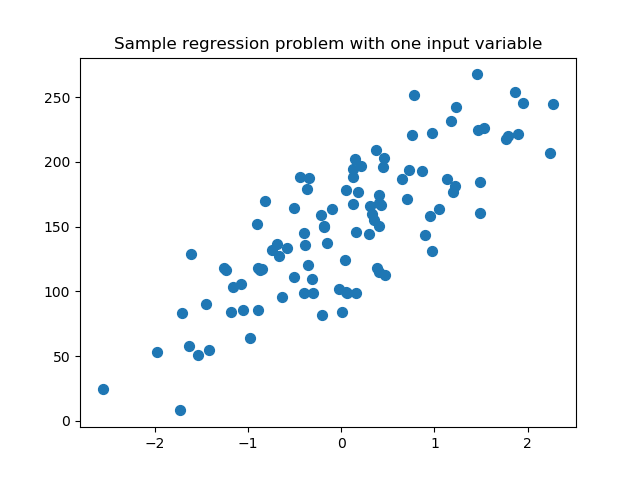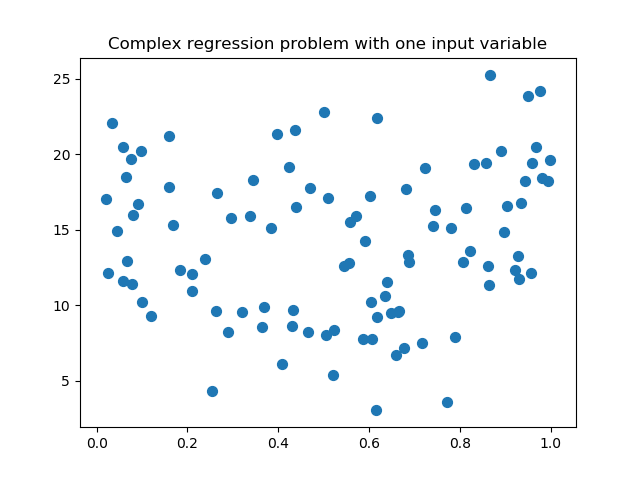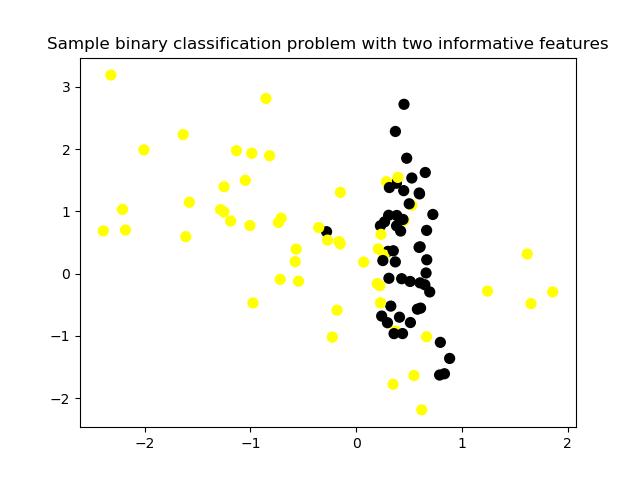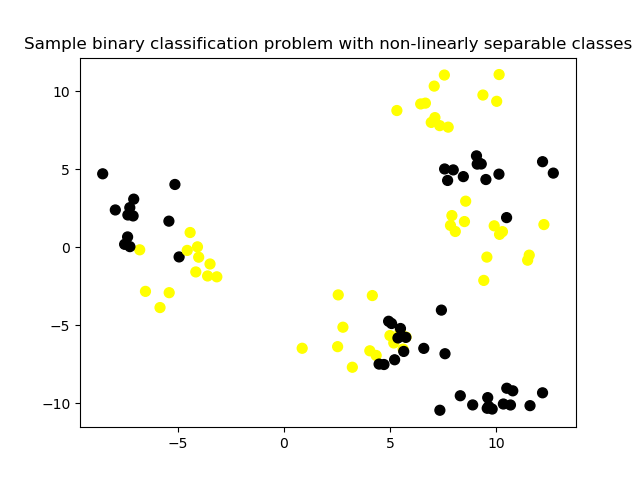## K-Nearest Neighbors¶

### Classification¶

In :
from adspy_shared_utilities import plot_two_class_knn

X_train, X_test, y_train, y_test = train_test_split(X_C2, y_C2,
random_state=0)

plot_two_class_knn(X_train, y_train, 1, 'uniform', X_test, y_test)
plot_two_class_knn(X_train, y_train, 3, 'uniform', X_test, y_test)
plot_two_class_knn(X_train, y_train, 11, 'uniform', X_test, y_test)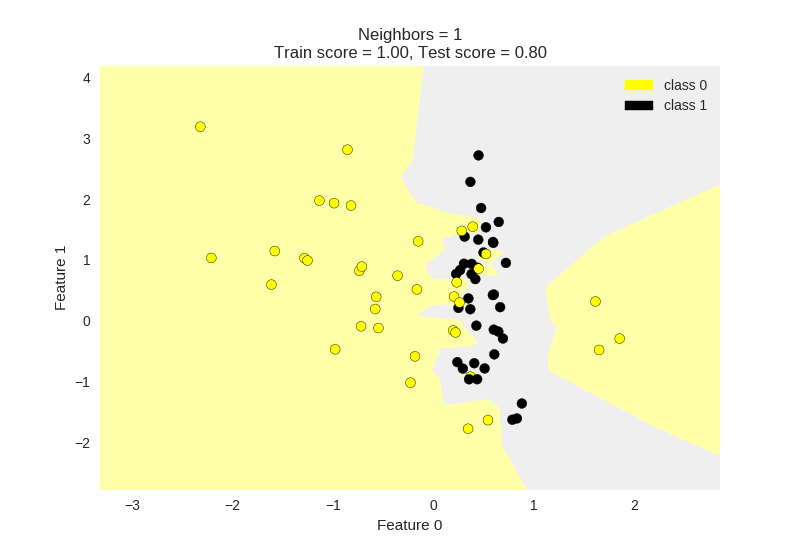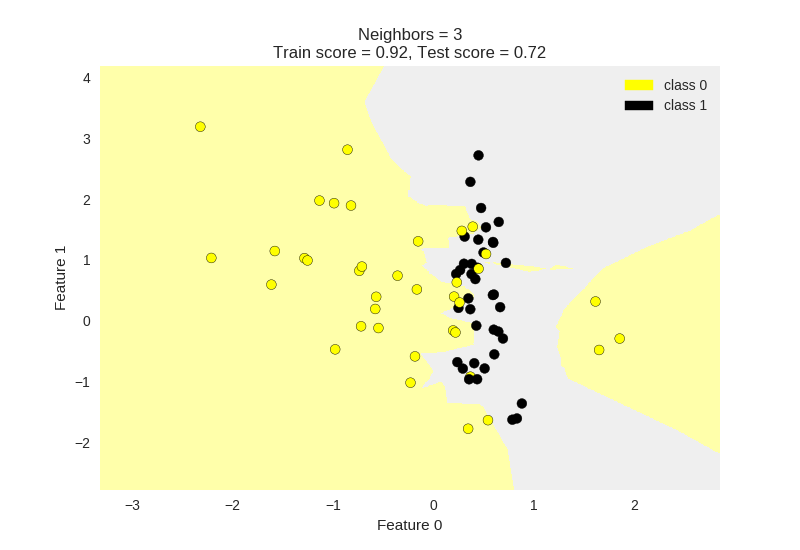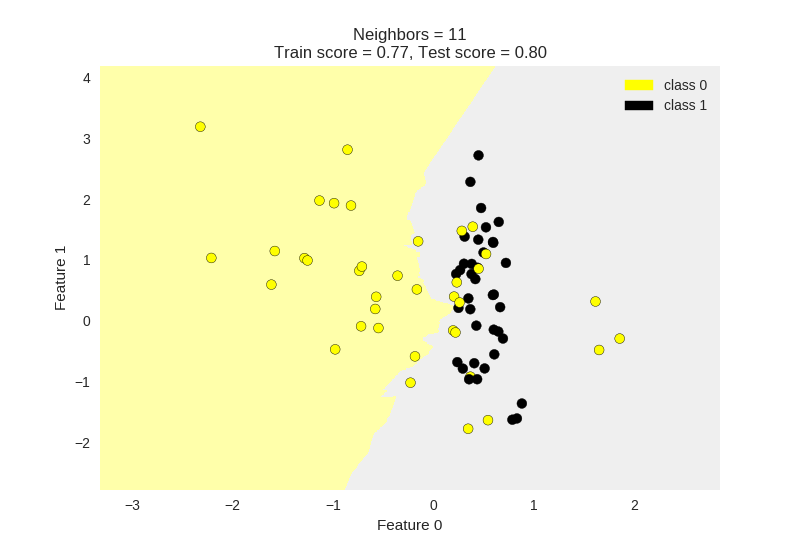### Regression¶

In :
from sklearn.neighbors import KNeighborsRegressor

X_train, X_test, y_train, y_test = train_test_split(X_R1, y_R1, random_state = 0)

knnreg = KNeighborsRegressor(n_neighbors = 5).fit(X_train, y_train)

print(knnreg.predict(X_test))
print('R-squared test score: {:.3f}'
.format(knnreg.score(X_test, y_test)))

[ 231.71  148.36  150.59  150.59   72.15  166.51  141.91  235.57  208.26
102.1   191.32  134.5   228.32  148.36  159.17  113.47  144.04  199.23
143.19  166.51  231.71  208.26  128.02  123.14  141.91]
R-squared test score: 0.425


## The $R^2$ (R-squared) regression score¶

• Measures how well a prediction model for regression fits the given data
• The score is between 0 and 1:
• A value of 0 corresponds to a constant model that predicts the mean value of all training target values.
• A values of 1 correspends to perfect prediction
• Also known as "coefficient of determination"
In :
fig, subaxes = plt.subplots(1, 2, figsize=(8,4))
X_predict_input = np.linspace(-3, 3, 50).reshape(-1,1)
X_train, X_test, y_train, y_test = train_test_split(X_R1[0::5], y_R1[0::5], random_state = 0)

for thisaxis, K in zip(subaxes, [1, 3]):
knnreg = KNeighborsRegressor(n_neighbors = K).fit(X_train, y_train)
y_predict_output = knnreg.predict(X_predict_input)
thisaxis.set_xlim([-2.5, 0.75])
thisaxis.plot(X_predict_input, y_predict_output, '^', markersize = 10,
label='Predicted', alpha=0.8)
thisaxis.plot(X_train, y_train, 'o', label='True Value', alpha=0.8)
thisaxis.set_xlabel('Input feature')
thisaxis.set_ylabel('Target value')
thisaxis.set_title('KNN regression (K={})'.format(K))
thisaxis.legend()
plt.tight_layout()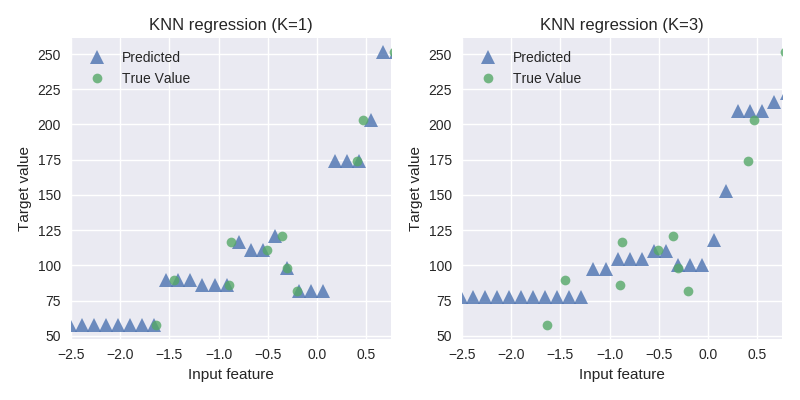### Regression model complexity as a function of K¶

In :
# plot k-NN regression on sample dataset for different values of K
fig, subaxes = plt.subplots(5, 1, figsize=(5,20))
X_predict_input = np.linspace(-3, 3, 500).reshape(-1,1)
X_train, X_test, y_train, y_test = train_test_split(X_R1, y_R1,
random_state = 0)

for thisaxis, K in zip(subaxes, [1, 3, 7, 15, 55]):
knnreg = KNeighborsRegressor(n_neighbors = K).fit(X_train, y_train)
y_predict_output = knnreg.predict(X_predict_input)
train_score = knnreg.score(X_train, y_train)
test_score = knnreg.score(X_test, y_test)
thisaxis.plot(X_predict_input, y_predict_output)
thisaxis.plot(X_train, y_train, 'o', alpha=0.9, label='Train')
thisaxis.plot(X_test, y_test, '^', alpha=0.9, label='Test')
thisaxis.set_xlabel('Input feature')
thisaxis.set_ylabel('Target value')
thisaxis.set_title('KNN Regression (K={})\n\
Train $R^2 = {:.3f}$,  Test $R^2 = {:.3f}$'
.format(K, train_score, test_score))
thisaxis.legend()